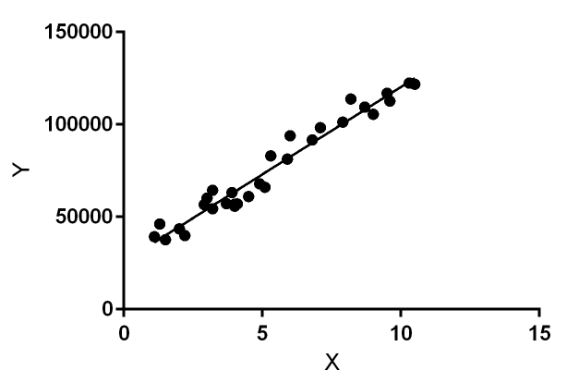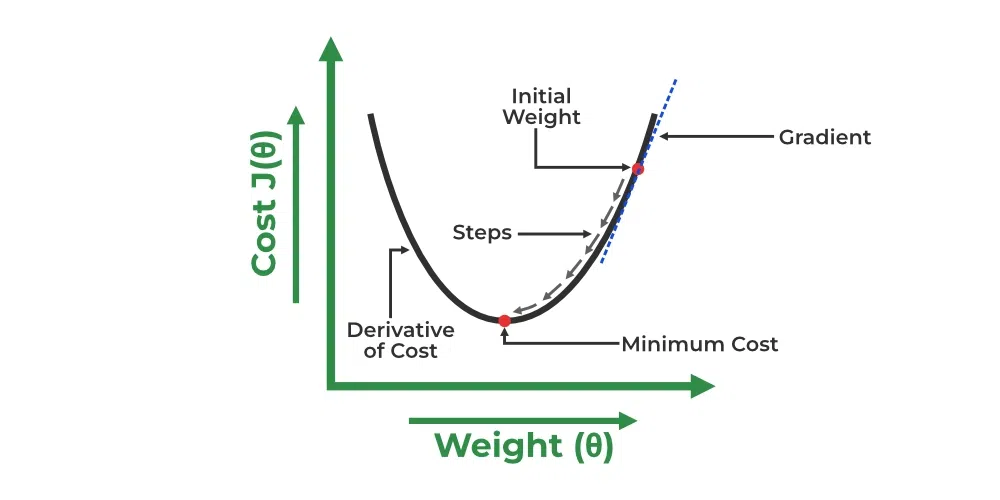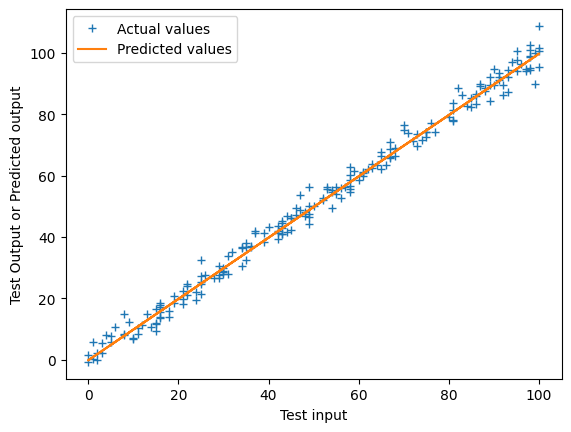GFG App
Open AppBrowser
Continue

# Linear Regression in Machine learning

Machine Learning is a branch of Artificial intelligence that focuses on the development of algorithms and statistical models that can learn from and make predictions on data. Linear regression is also a type of machine-learning algorithm more specifically a supervised machine-learning algorithm that learns from the labeled datasets and maps the data points to the most optimized linear functions. which can be used for prediction on new datasets.

First of we should know what is supervised machine learning algorithms. It is a type of machine learning where the algorithm learns from labeled data.  Labeled data means the dataset whose respective target value is already known. Supervised learning has two types:

• Classification: It predicts the class of the dataset based on the independent input variable. Class is the categorical or discrete values. like the image of an animal is a cat or dog?
• Regression: It predicts the continuous output variables based on the independent input variable. like the prediction of house prices based on different parameters like house age, distance from the main road, location, area, etc.

Here, we will discuss one of the simplest types of regression i.e Linear Regression.

## Linear Regression

Linear regression is a type of supervised machine learning algorithm that computes the linear relationship between a dependent variable and one or more independent features. When the number of the independent feature, is 1 then it is known as Univariate Linear regression, and in the case of more than one feature, it is known as multivariate linear regression. The goal of the algorithm is to find the best linear equation that can predict the value of the dependent variable based on the independent variables. The equation provides a straight line that represents the relationship between the dependent and independent variables. The slope of the line indicates how much the dependent variable changes for a unit change in the independent variable(s).

Linear regression is used in many different fields, including finance, economics, and psychology, to understand and predict the behavior of a particular variable. For example, in finance, linear regression might be used to understand the relationship between a company’s stock price and its earnings or to predict the future value of a currency based on its past performance.

One of the most important supervised learning tasks is regression. In regression set of records are present with X and Y values and these values are used to learn a function so if you want to predict Y from an unknown X this learned function can be used. In regression we have to find the value of Y, So, a function is required that predicts continuous Y in the case of regression given X as independent features.

Here Y is called a dependent or target variable and X is called an independent variable also known as the predictor of Y. There are many types of functions or modules that can be used for regression. A linear function is the simplest type of function. Here, X may be a single feature or multiple features representing the problem.Linear Regression

Linear regression performs the task to predict a dependent variable value (y) based on a given independent variable (x)). Hence, the name is Linear Regression. In the figure above, X (input) is the work experience and Y (output) is the salary of a person. The regression line is the best-fit line for our model.

### Assumption for Linear Regression Model

Linear regression is a powerful tool for understanding and predicting the behavior of a variable, however, it needs to meet a few conditions in order to be accurate and dependable solutions.

1. Linearity: The independent and dependent variables have a linear relationship with one another. This implies that changes in the dependent variable follow those in the independent variable(s) in a linear fashion.
2. Independence: The observations in the dataset are independent of each other. This means that the value of the dependent variable for one observation does not depend on the value of the dependent variable for another observation.
3. Homoscedasticity: Across all levels of the independent variable(s), the variance of the errors is constant. This indicates that the amount of the independent variable(s) has no impact on the variance of the errors.
4. Normality: The errors in the model are normally distributed.
5. No multicollinearity: There is no high correlation between the independent variables. This indicates that there is little or no correlation between the independent variables.

### Hypothesis function for Linear Regression :

As we have assumed earlier that our independent feature is the experience i.e X and the respective salary Y is the dependent variable. Let’s assume there is a linear relationship between X and Y then the salary can be predicted using:Here,

•are labels to data (Supervised learning)
•are the input independent training data (univariate – one input variable(parameter))
•are the predicted values.

The model gets the best regression fit line by finding the best θ1 and θ2 values.

• θ1: intercept
• θ2: coefficient of x

Once we find the best θ1 and θ2 values, we get the best-fit line. So when we are finally using our model for prediction, it will predict the value of y for the input value of x.

#### Cost function

The cost function or the loss function is nothing but the error or difference between the predicted valueand the true value Y. It is the Mean Squared Error (MSE) between the predicted value and the true value. The cost function (J) can be written as:To achieve the best-fit regression line, the model aims to predict the target valuesuch that the error difference between the predicted valueand the true value Y is minimum. So, it is very important to update the θ1 and θ2 values, to reach the best value that minimizes the error between the predicted y value (pred) and the true y value (y).A linear regression model can be trained using the optimization algorithm gradient descent by iteratively modifying the model’s parameters to reduce the mean squared error (MSE) of the model on a training dataset. To update θ1 and θ2 values in order to reduce the Cost function (minimizing RMSE value) and achieve the best-fit line the model uses Gradient Descent. The idea is to start with random θ1 and θ2 values and then iteratively update the values, reaching minimum cost.

A gradient is nothing but a derivative that defines the effects on outputs of the function with a little bit of variation in inputs.

Let’s differentiate the cost function(J) with respect toLet’s differentiate the cost function(J) with respect toFinding the coefficients of a linear equation that best fits the training data is the objective of linear regression. By moving in the direction of the Mean Squared Error negative gradient with respect to the coefficients, the coefficients can be changed. And the respective intercept and coefficient of X will be ifis the learning rate.## Python3

 import pandas as pd import numpy as np import matplotlib.pyplot as plt import matplotlib.axes as ax

## Python3

 data = pd.read_csv('data_for_lr.csv')   # Drop the missing values data = data.dropna()   # training dataset and labels train_input = np.array(data.x[0:500]).reshape(500,1) train_output  = np.array(data.y[0:500]).reshape(500,1)   # valid dataset and labels test_input = np.array(data.x[500:700]).reshape(199,1) test_output  = np.array(data.y[500:700]).reshape(199,1)

#### Steps:

• In forward propagation, Linear regression function Y=mx+x is applied by initially assigning random value of parameter (m & c).
• The we have written the function to finding the cost function i.e the mean

## Python3

 class LinearRegression:     def __init__(self):         self.parameters = {}           def forward_propagation(self, train_input):         m = self.parameters['m']         c = self.parameters['c']         predictions = np.multiply(m, train_input) + c         return predictions       def cost_function(self, predictions, train_output):         cost = np.mean((train_output - predictions) ** 2)         return cost       def backward_propagation(self, train_input, train_output, predictions):         derivatives = {}         df = (train_output - predictions) * -1         dm = np.mean(np.multiply(train_input, df))         dc = np.mean(df)         derivatives['dm'] = dm         derivatives['dc'] = dc         return derivatives       def update_parameters(self, derivatives, learning_rate):         self.parameters['m'] = self.parameters['m'] - learning_rate * derivatives['dm']         self.parameters['c'] = self.parameters['c'] - learning_rate * derivatives['dc']       def train(self, train_input, train_output, learning_rate, iters):         #initialize random parameters         self.parameters['m'] = np.random.uniform(0,1) * -1         self.parameters['c'] = np.random.uniform(0,1) * -1                   #initialize loss         self.loss = []                   #iterate         for i in range(iters):             #forward propagation             predictions = self.forward_propagation(train_input)               #cost function             cost = self.cost_function(predictions, train_output)               #append loss and print             self.loss.append(cost)             print("Iteration = {}, Loss = {}".format(i+1, cost))               #back propagation             derivatives = self.backward_propagation(train_input, train_output, predictions)               #update parameters             self.update_parameters(derivatives, learning_rate)           return self.parameters, self.loss

## Python3

 #Example usage linear_reg = LinearRegression() parameters, loss = linear_reg.train(train_input, train_output, 0.0001, 20)

Output:

Iteration = 1, Loss = 5363.981028641572
Iteration = 2, Loss = 2437.9165904342512
Iteration = 3, Loss = 1110.3579137897523
Iteration = 4, Loss = 508.043071737168
Iteration = 5, Loss = 234.7721607488976
Iteration = 6, Loss = 110.78884574712548
Iteration = 7, Loss = 54.53747840152165
Iteration = 8, Loss = 29.016170730218153
Iteration = 9, Loss = 17.43712517102535
Iteration = 10, Loss = 12.183699375121314
Iteration = 11, Loss = 9.800214272338595
Iteration = 12, Loss = 8.718824440889573
Iteration = 13, Loss = 8.228196676299069
Iteration = 14, Loss = 8.005598315794709
Iteration = 15, Loss = 7.904605192804647
Iteration = 16, Loss = 7.858784500769819
Iteration = 17, Loss = 7.837995601770647
Iteration = 18, Loss = 7.828563654998014
Iteration = 19, Loss = 7.824284370030002
Iteration = 20, Loss = 7.822342853430061

## Python3

 #Prediction on test data y_pred = test_input*parameters['m'] + parameters['c']   # Plot the regression line with actual data pointa plt.plot(test_input, test_output, '+', label='Actual values') plt.plot(test_input, y_pred, label='Predicted values') plt.xlabel('Test input') plt.ylabel('Test Output or Predicted output') plt.legend() plt.show()

Output:Best fit Linear regression line with actual values

My Personal Notes arrow_drop_up